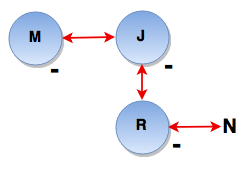# Blood Relations and Family Tree - Logical Reasoning (MCQ) Questions for Q. 29955

1. P x Q means P is the father of Q
2. P - Q means P is the sister of Q
3. P + Q means P is the mother of Q
4. P ÷ Q means P is the brother of Q

Which of the following represents ‘R is niece of M’?

- Published on 08 May 17

a. M – J + R – N
b. R – M x T - W
c. M – K x T - R
d. None of these

ANSWER: M – J + R – N

R is the niece of M means R is the daughter of brother or sister of M i.e. M – J + R – N or M – J x R – N#### Discussion

• sitnanshu jadhav    -Posted on 31 Jan 22
op man and nice ques

## ➨ Post your comment / Share knowledgeEnter the code shown above:

(Note: If you cannot read the numbers in the above image, reload the page to generate a new one.)